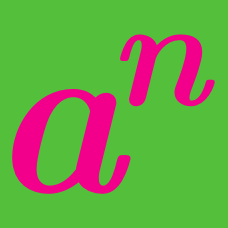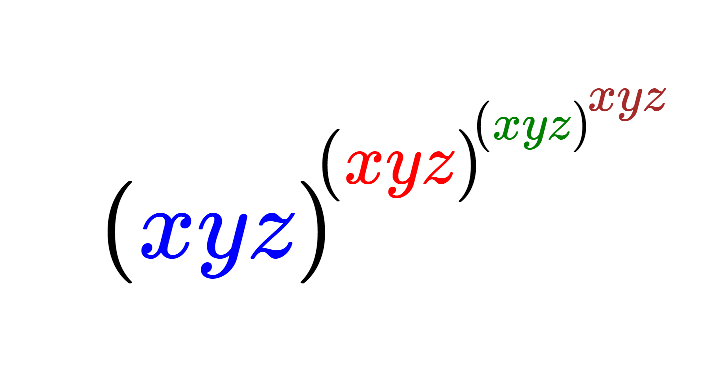Algebra

# Exponential Functions: Level 2 Challenges

$\large 2^x=\dfrac{1}{2^y}, \quad\quad\quad x + y = \ ?$

$\large 2^{x} = 3^{y} = 12^{z}$

If the equation above is fulfilled for non-zero values of $x,y,z,$ find the value of $\frac { z(x+2y) }{ xy }$.

$\large{ \begin{cases} ab = a^b \\\\ \frac{a}{b} = a ^ {3b} \\ \end{cases}}$

$a$ and $b$ are real numbers such that $a > 1$ and $b \neq 0$, satisfying the above system.

Find $b ^ { -a}.$

$\large 5^{2x}+5^5=5^{x+3}+5^{x+2}$

Find the sum of the solutions of $x$ that satisfy the equation above.Given that $x,y,z$ are positive real numbers that satisfy the equations: $x = y^z, y=z^x, z = x^y$, find the value of the expression above.

×